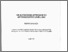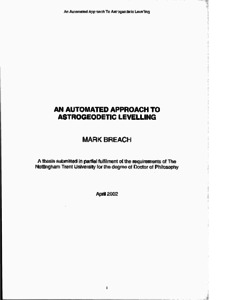# An automated approach to astrogeodetic levelling

Breach, M., 2002. An automated approach to astrogeodetic levelling. PhD, Nottingham Trent University.Preview
Text
10183507.pdf - Published version

## Abstract

Height determined using the Global Positioning System (GPS) is height above the World Geodetic System 1984 ellipsoid, a mathematical model of the earth. Surveyors and engineers require orthometric height, normally realized as height above sea level. Therefore knowledge of "separation'' between height systems is essential when using GPS for the precise determination of orthometric height. One method of finding separation is by "Astrogeodetic Levelling".

Astronomic position may be determined by "Position Line" observations to stars and geodetic position by GPS. The difference between astronomic and geodetic positions, "deviation of the vertical', is the same as the rate of change of separation.

In this research a least squares approach to Position Lines was developed, in which refraction, vertical collimation and their rates of change are modelled as unknowns. Equations to correct for the effect on the observed vertical angle of an error in horizontal pointing were developed. Observing and computing strategies were considered. Star catalogues were constructed and tested. The effect of lunar gravitation and barycentric centrifugal force on deviation of the vertical was examined and new theory was developed to correct for this source of error. Several possible solutions to the evaluation of a personal equation were examined. A simple method to determine the topographic-isostatic effect using wedges across a longitudinal section on the line of greatest slope through a point was developed.

Video-based methods for detecting the instant of passage of a star across theodolite crosshairs and for linking GPS and video time by exposure of a GPS timed flash were developed. Analysis of the Astrogeodetic Geoid Model using ideas based on Kaula's "rule of thumb" was made and the geoid was modelled by polynomial coefficients and by interpolation of deviations. This leads to the application of "progressive nodes" as a means of avoiding a full least squares solution.

A practical determination of astronomical position was made giving position to about 0".15 (5 metres) with less than an hour's worth of observations. This suggests that astronomically derived deviation is an economical data source for the determination of a local precise geoid model. The process may find a practical application where precise height is required from GPS, such as in large engineering projects, especially where a precise relative local geoid model is only required in the area of the works. In this case, an astrogeodetic geoid will be much easier to construct than a gravimetrically derived one because it will require fewer observations.

Item Type: Thesis
Creators: Breach, M.
Date: 2002
ISBN: 9781369316711
Identifiers:
NumberType
PQ10183507Other
Divisions: Schools > School of Architecture, Design and the Built Environment
Record created by: Linda Sullivan
Date Added: 30 Sep 2020 12:48Edit View